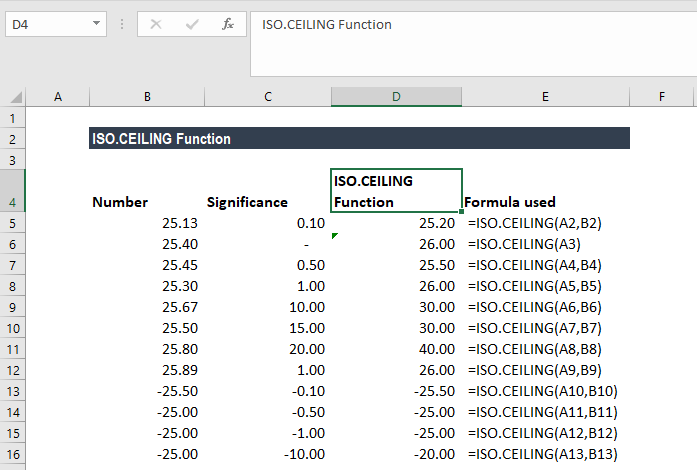# ISO.CEILING Function

Returns a number rounded up to the nearest integer or multiple of significance

## What is the ISO.CEILING Function?

The ISO.CEILING function is an Excel Math and Trigonometry function. It is one of the fifteen rounding functions of MS Excel. The function returns a number rounded up to the nearest integer or to the nearest multiple of significance. It works regardless of whether the number given is positive or negative.

ISO.CEILING was introduced in MS Excel 2010 and hence is unavailable in earlier versions.

### Formula

=ISO.CEILING(number,[significance])

The ISO.CEILING function uses the following arguments:

1. Number (required argument) – This is the value that to be rounded.
2. Significance (optional argument) – This is the optional multiple to which the number given will be rounded.

### How to use the ISO.CEILING Function in Excel?

To understand the uses of the ISO.CEILING function, let’s consider an example:

#### Example

As shown below, we took the number range -25 to 25.90 and used different significance values.From the results above, we can observe that:

1. When we gave the significance as 0.10, the function rounded off 25.13 to 25.2, which is the farthest 0.
2. In the second example, we left the argument significance as blank so the function used 1.
3. In the third example, we gave the significance as 0.5, so it rounded off to the nearest 0.5, which is 25.5. If the number is 25.55, it will round it off to 26.
4. When we provided the significance as 1, it rounded it off to 26. Even if we provided 25.7, the function would round it off to 26. This is so as the function will round it off to the nearest multiple of 1.
5. For rows 9, 10 and 11, the function rounded off the number to the nearest multiple of 10, 15, and 20.
6. In row 13, when we gave the significance as -0.10, the function rounded 25.5 up to the nearest multiple of -0.1.

### A few notes about the ISO.CEILING Function

1. If we omit the [significance] argument, it will take on the default value of 1.
2. The ISO.CEILING function always rounds the supplied number up. For example, if the number given is positive, the function will round it up away from zero, and if the number given is negative, it will round it up towards zero.
3. #VALUE! error – Occurs when we provide non-numeric values for one or both of the arguments.

Thanks for reading CFI’s guide to important Excel functions! By taking the time to learn and master these functions, you’ll significantly speed up your financial analysis. To learn more, check out these additional CFI resources:

• Excel Functions for Finance